Electron. J. Diff. Equ., Vol. 2015 (2015), No. 311, pp. 1-13.

### Quenching behavior of semilinear heat equations with singular boundary conditions Burhan Selcuk, Nuri Ozalp

Abstract:
In this article, we study the quenching behavior of solution to the semilinear heat equation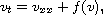with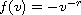or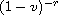and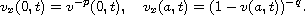For this, we utilize the quenching problem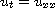with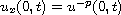,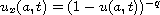. In the second problem, if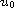is an upper solution (a lower solution) then we show that quenching occurs in a finite time, the only quenching point is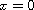() and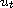blows up at quenching time. Further, we obtain a local solution by using positive steady state. In the first problem, we first obtain a local solution by using monotone iterations. Finally, for(), if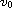is an upper solution (a lower solution) then we show that quenching occurs in a finite time, the only quenching point is() and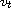blows up at quenching time.

Submitted October 16, 2015. Published December 21, 2015.
Math Subject Classifications: 35K05, 35K15, 35B50.
Key Words: Heat equation; singular boundary condition; quenching; maximum principle; monotone iteration.

Show me the PDF file (239 KB), TEX file, and other files for this article.

 Burhan Selcuk Department of Computer Engineering Karabuk University Bali klarkayasi Mevkii 78050, Turkey email: bselcuk@karabuk.edu.tr, burhanselcuk44@gmail.com Nuri Ozalp Department of Mathematics, Ankara University Besevler 06100, Turkey email: nozalp@science.ankara.edu.tr